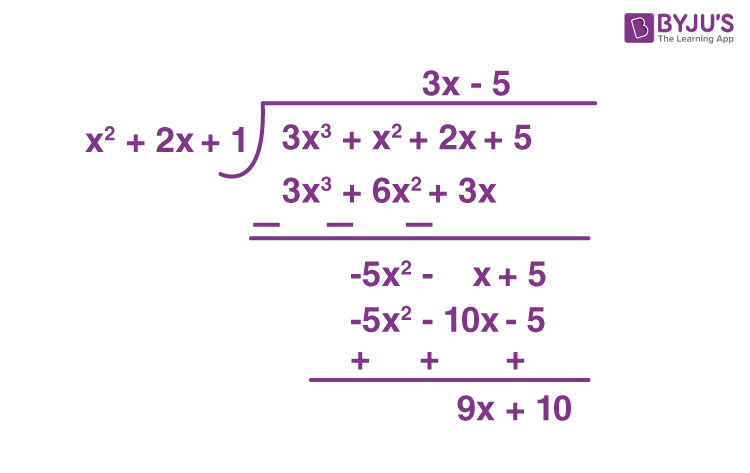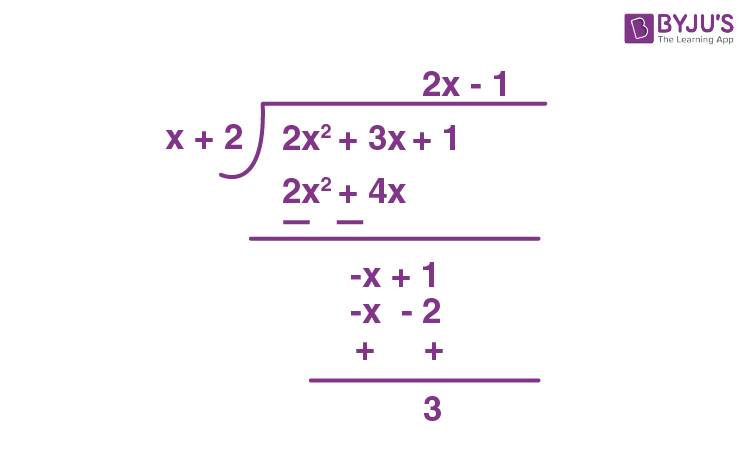# Division Algorithm for Polynomials

We know that a polynomial expression is an algebraic expression, which is made up of variables, coefficients, terms and constants and mathematical operators. We have learned that we can apply various arithmetic operations to real numbers. Similarly, we can also perform addition, subtraction, multiplication and division operations on polynomials. In this article, we are going to learn the “Division Algorithm for Polynomials” with solved examples.

## Division Algorithm for Polynomials Statement

The division algorithm for polynomials states that, if p(x) and g(x) are any two polynomials with g(x) ≠ 0, then we can find polynomials q(x) and r(x) such that

p(x) = g(x) × q(x) + r(x)

where r(x) = 0 or degree of r(x) < degree of g(x).

Here,

p(x) represents the dividend polynomial

g(x) represents the divisor polynomial

q(x) represents the quotient polynomial

r(x) represents the remainder polynomial

### Division Algorithm for Polynomials Example

Go through the below-provided example to understand the division algorithm for polynomials, which is given in step by step procedure.

Example 1:

Divide the cubic polynomial 3x3+x2+2x+5 by the quadratic polynomial 1+2x+x2.

Solution:

Given:

Dividend = 3x3+x2+2x+5

Divisor = 1+2x+x2

Step 1: Arrange the terms of the dividend and divisor polynomial in the decreasing order of their degrees.

Therefore, dividend = 3x3+x2+2x+5, divisor = x2+2x+1.

Step 2: To get the first term of the quotient, divide the highest degree term of the dividend (3x3) by the highest degree term of the divisor (x2), which results in the quotient 3x, and carry out the division process. Hence, the remainder obtained in the first step of the division process is -5x2-x+5.

Step 3: Now, to get the second term of the quotient, divide the first term of the new dividend (i.e) -5x2-x+5, by the highest degree term of the divisor (i.e., x2). Hence, the second term of the quotient obtained is -5 and continues the division process with -5x2-x+5. Hence, the remainder obtained in the second step of the division process is 9x+10. Since the degree of the remainder (9x+10) is less than the degree of the divisor ( x2+2x+1), we cannot continue the division process further.

Hence, quotient = 3x-5 and remainder is 9x+10.Verification:

We know that, Dividend = Divisor × Quotient + Remainder

3x3+x2+2x+5 =(x2+2x+1)(3x-5)+(9x+10)

3x3+x2+2x+5 = 3x3+6x2+3x-5x2-10x-5+9x+10

3x3+x2+2x+5 = 3x3+x2+2x+5

Therefore, L.H.S = R.H.S

Hence, the quotient = 3x+5 and remainder = 9x+10.

Example 2:

Divide the quadratic polynomial 2x2+3x+1 by the linear polynomial x+2

Solution:

Here, the dividend is 2x2+3x+ 1 and the divisor is x+2.Here, the remainder is 3, which is equal to 3x0. [(i.e) The degree of 3 is less than the degree of x+2].

Therefore, if 2x2+3x+1 is divided by x+2, the quotient obtained is 2x-1 and the remainder obtained is 3.

(Note: We have to stop the division process if the remainder is either 0 or the degree of the remainder is less than the degree of the divisor).

### Practice Problems

Solve the following problems.

1. Divide the polynomial 3x2-x3-3x+5 by -x2+x-1 and verify it using the division algorithm for polynomials.
2. Divide the polynomial x4-5x+6 by 2-x2 and find its quotient and remainder.
3. Find q(x) and r(x), if p(x)=x4-3x2+4x+5 and g(x) = x2-x+1.

Keep visiting BYJU’S – The Learning App and download the app to learn all Maths-related articles easily by exploring more videos.